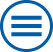## The group contributes to the following mathematical research topics of WIAS:

Systems of partial differential equations: modeling, numerical analysis and simulation

The mathematical description of many scientific and technological problems leads to systems of partial differential equations (PDEs). [>> more]